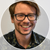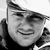# App Development

Discussion board where members can learn more about Qlik Sense App Development and Usage.

Announcements
Welcome to Qlik Community! Check out our new navigation! FIND OUT MORE
cancel
Showing results for
Did you mean:Partner - Contributor III

## Calculation condition possible values, multiple values

I want to be able to easily add and subtract fields from possible values in a calculation condition. If there is any other combination I don't want it to be true.  In the example below I get it to work with two values; Value1 and Value2.

if(concat(distinct [Field1], ', ') = 'Value1' or
concat(distinct[Field1], ', ') ='Value2' or
concat(distinct[Field1], ', ') ='Value1, Value2',1,0)

I want to be able to do it easily for more than 2 values. For each value added the code would get longer, the next example is with a third added value;

if(concat(distinct [Field1], ', ') = 'Value1' or
concat(distinct[Field1], ', ') ='Value2' or
concat(distinct[Field1], ', ') ='Value3' or
concat(distinct[Field1], ', ') ='Value1, Value2' or
concat(distinct[Field1], ', ') ='Value1, Value3' or
concat(distinct[Field1], ', ') ='Value2, Value3' or
concat(distinct[Field1], ', ') ='Value1, Value2, Value3',1,0)

So the code will get exponentially longer and longer, even though I just want another field value added. Has anyone done anything similar without the hassle of longer and longer code?

Labels (4)

• ### if

1 Solution

Accepted SolutionsMVP

What if you do a comparison like the one I've done below? In my quick test it seems to work as desired.

``````if(
Concat(DISTINCT Field1, ', ')
=
Concat({< Field1 *= {'Value1', 'Value2'}>}DISTINCT Field1, ', ')
,1,0)``````

I've attached my test qvw app

14 RepliesMVP

``````If(
SubStringCount(Concat(DISTINCT Field1, ', '), 'Value1') = 1 or
SubStringCount(Concat(DISTINCT Field1, ', '), 'Value2') = 1 or
SubStringCount(Concat(DISTINCT Field1, ', '), 'Value3') = 1
, 1, 0)``````MVP

You can also do this with WildMatch or Like function.

``````If(
WildMatch(Concat(DISTINCT Field1, ', '), '*Value1*', '*Value2*', '*Value3*')
, 1, 0)``````Partner - Contributor III
Author

This would mean that if there are other possible values then it will still get calculated. For example if it's possible to choose Value1 and Value5MVP

I guess so... but isn't that what you original expression will do to?Partner - Contributor III
Author

No, it will exclude everything that isn't exactly just 1, just 2 or just 3 or any combination of the threeMVP

Right, that's true. I got confused. Let me think about itMVP

This is still not perfect, but see if this looks any better

``````=If(GetSelectedCount(Field1) = 1,
If(Match(Concat(DISTINCT Field1, ', '), 'Value1', 'Value2', 'Value3'), 1, 0),
If(GetSelectedCount(Field1) = 2,
If(Match(Concat(DISTINCT Field1, ', '), 'Value1, Value2', 'Value1, Value3', 'Value2, Value3'), 1, 0),
If(GetSelectedCount(Field1) = 3,
If(Match(Concat(DISTINCT Field1, ', '), 'Value1, Value2, Value3'), 1, 0), 0)))``````Partner - Contributor III
Author

It gets the same job done, but the underlying problem remains. If I would add a Value4 it would get larger, and then a Value5, even larger than that. Thanks for the help and your time but the problem remainsMVP

I agree... the only way to make this easy is to create a new field in the script

``````Table:
If(Match(Field1, 'Value1', 'Value2', 'Value3'), 1, 0) as Flag;
LOAD * INLINE [
Field1
Value1
Value2
Value3
Value4
Value5
];``````

And use this expression

``=Min(Flag)``

This should work just like your actual expressionTags
Community Browser